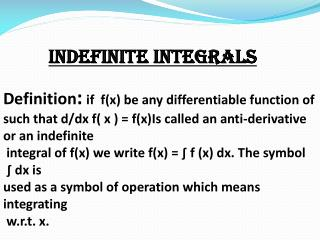DownloadDownload PresentationIndefinite integrals

# Indefinite integrals

Télécharger la présentation## Indefinite integrals

- - - - - - - - - - - - - - - - - - - - - - - - - - - E N D - - - - - - - - - - - - - - - - - - - - - - - - - - -
##### Presentation Transcript

1. Indefinite integrals Definition:if f(x) be any differentiable function of such that d/dx f( x ) = f(x)Is called an anti-derivative or an indefinite integral of f(x) we write f(x) = ∫ f (x) dx. The symbol ∫ dx is used as a symbol of operation which means integrating w.r.t. x.

2. In all the above formulas, c is the constant of integration. Some standard results on integration 1.∫ k f(x) dx = k ∫ f(x) dx, where k is a constant 2. ∫ ( f(x) ± g(x))dx = ∫ f(x)dx ± ∫ g(x)dx

3. Example: ∫4x⁷ dx =4∫x⁷ dx =4∫xⁿ⁺¹/n+1 =4 x⁸/ + c = x⁸/8+ c 8

4. (1) ∫(sinx + cosx) dx = ∫sinxdx + ∫cosxdx = -cosx + sinx + C

5. METHODS OF INTEGRATION It was based on inspection, i.e,. On the search of a function F whose derivative is f which led us to the integral of f . However, this method, which depends on inspection , is not very suitable for many functions. Hence, we need to develop additional techniques or methods for finding the integrals by reducing Them into standard forms.

6. Following are the method of integration: Integration by substitution Integration by part Integration by partial fractions

7. Integration by substitution This method is used when basic rules of Integration given above, are not directly applicable. In such cases, the integration is transformed (by suitable substitution of given variable by a new variable) into a form to which basic rules of integration are applicable

8. Equation of integrals (a) ∫ √15 + logx dx x put 15 + logx = t 1/x dx = dt Substituting in given question, we get ∫√t dt = ∫t½ dt = 2/3t³⁄² + C

9. Conti…… = 2/3 (15 + logx)³⁄² + C Where c = Constant of integration.

10. Integration by parts This method is based on product rule differentiation. According to this method, the integral of product of Two functions f(x). g(x) is given by ∫ g(x) dx - f ’(x) ( g(x)dx)dx ∫ ∫ ∫ F(x) . g(x) dx = f(x)

11. Taking f(x) as the first function (I) and g(x) as the second (II) the above equation can be Stated as ∫I . II dx = I∫IIdx - ∫(d(I)/dx) dx

12. Rule for the proper chose of the first function The first function to be selected may be the one which comes first in the order ILATE where I means inverse trigonometric function L means logarithmic function A means algebraic T means trigonometric E means exponential.

13. ∫x² e dx x • x Take x² as the function and e as the second function and integrating by parts, we get I = x² e - ∫2x e x X dx

14. Again apply by parts in second term, we get x x I = x² e – 2 [xe - ∫e dx] X X = x² e – 2xe + 2e + C, Where c.is the constant of integration X

15. Integration by partial fraction If f(x) and g(x) are two polynomials, then f(x)/g(x) is called a rational algebraic Function of x. Any rational f (x)/ g(x) can be expressed as the sum of rational functions each having a simple factor of g(x). Each such fraction is called a partial fraction.

16. 3x 1. ∫ dx (x-1) (x+2) A B = + X-1 X + 2 3x = A (X + 2) + B (X + 1)

17. Put,, X = 1 we get 3 = 3A A = 1 Put, x = -2 we get - 6 = - 3B B = 2

18. 3x (x+2) (x-1) 1 2 = + X-1 X + 2 Now integrating, we get 3x 2 1 X - 1 ∫ ∫ dx = ∫ dx+ 2 ( x - 1 ) ( x + 2) X + 2

19. = log ( x – 1 ) + 2 log ( x + 2 ) + c

20. Thank you Presented by, NUZHAT SHAHEEN